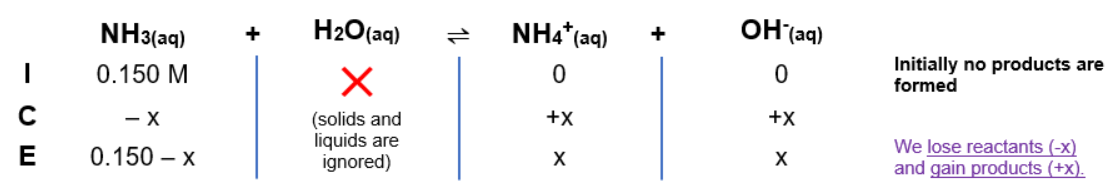# Problem: What are the major species present in a 0.150-M NH 3 solution? Calculate the [OH -] and the pH of this solution.

###### FREE Expert Solution

Equilibrium reaction:        NH3 (aq) + H2O(l)  NH4+(aq) + OH-(aq)

$\overline{){{\mathbf{K}}}_{{\mathbf{b}}}{\mathbf{=}}\frac{\mathbf{products}}{\mathbf{reactants}}}\phantom{\rule{0ex}{0ex}}{\mathbf{K}}_{\mathbf{b}}\mathbf{=}\frac{\left[{{\mathbf{NH}}_{\mathbf{4}}}^{\mathbf{+}}\right]\left[{\mathbf{OH}}^{\mathbf{-}}\right]}{\left[{\mathbf{NH}}_{\mathbf{4}}\right]}$

Solids and liquids are not included in the expression

ICE CHART###### Problem Details

What are the major species present in a 0.150-M NH 3 solution? Calculate the [OH -] and the pH of this solution.